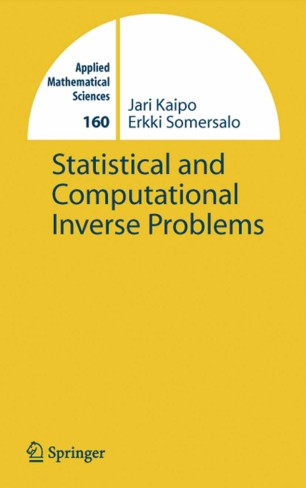# Statistical and Computational Inverse Problems

• Jari P. Kaipio
• Erkki SomersaloBook

Part of the Applied Mathematical Sciences book series (AMS, volume 160)

1. Front Matter
Pages I-XVI
2. Pages 7-48
3. Pages 49-114
4. Pages 115-143
5. Pages 145-188
6. Pages 189-221
7. Pages 223-309
8. Back Matter
Pages 311-339

### Introduction

The book develops the statistical approach to inverse problems with an emphasis on modeling and computations. The framework is the Bayesian paradigm, where all variables are modeled as random variables, the randomness reflecting the degree of belief of their values, and the solution of the inverse problem is expressed in terms of probability densities. The book discusses in detail the construction of prior models, the measurement noise modeling and Bayesian estimation. Markov Chain Monte Carlo-methods as well as optimization methods are employed to explore the probability distributions. The results and techniques are clarified with classroom examples that are often non-trivial but easy to follow. Besides the simple examples, the book contains previously unpublished research material, where the statistical approach is developed further to treat such problems as discretization errors, and statistical model reduction. Furthermore, the techniques are then applied to a number of real world applications such as limited angle tomography, image deblurring, electrical impedance tomography and biomagnetic inverse problems. The book is intended to researchers and advanced students in applied mathematics, computational physics and engineering. The first part of the book can be used as a text book on advanced inverse problems courses.

The authors Jari Kaipio and Erkki Somersalo are Professors in the Applied Physics Department of the University of Kuopio, Finland and the Mathematics Department at the Helsinki University of Technology, Finland, respectively.

### Keywords

Markov Measure Probability distribution Random variable biomedical engineering construction mechanics model modeling optimization

#### Authors and affiliations

• Jari P. Kaipio
• 1
• Erkki Somersalo
• 2
1. 1.Department of Applied PhysicsUniversity of KuopioKuopioFinland
2. 2.Institute of MathematicsHelsinki University of TechnologyFinland

### Bibliographic information

• DOI https://doi.org/10.1007/b138659